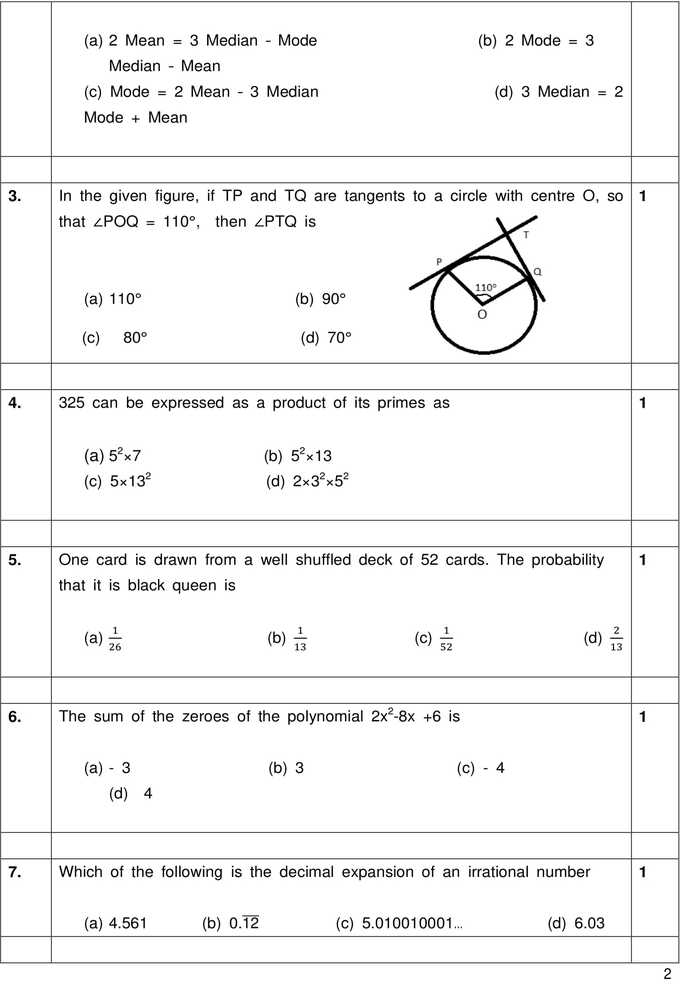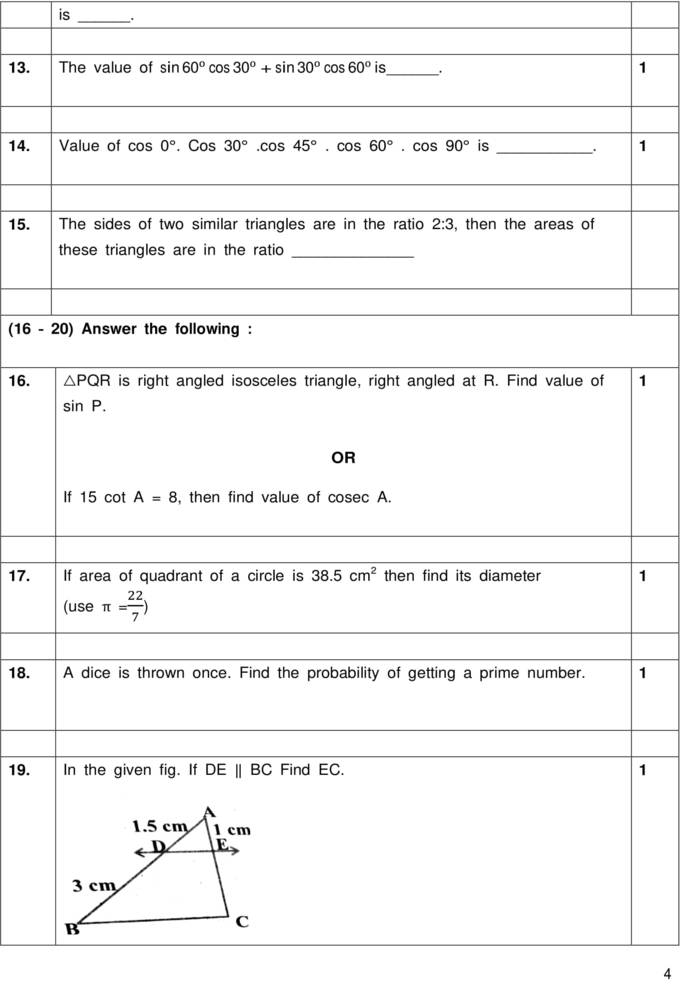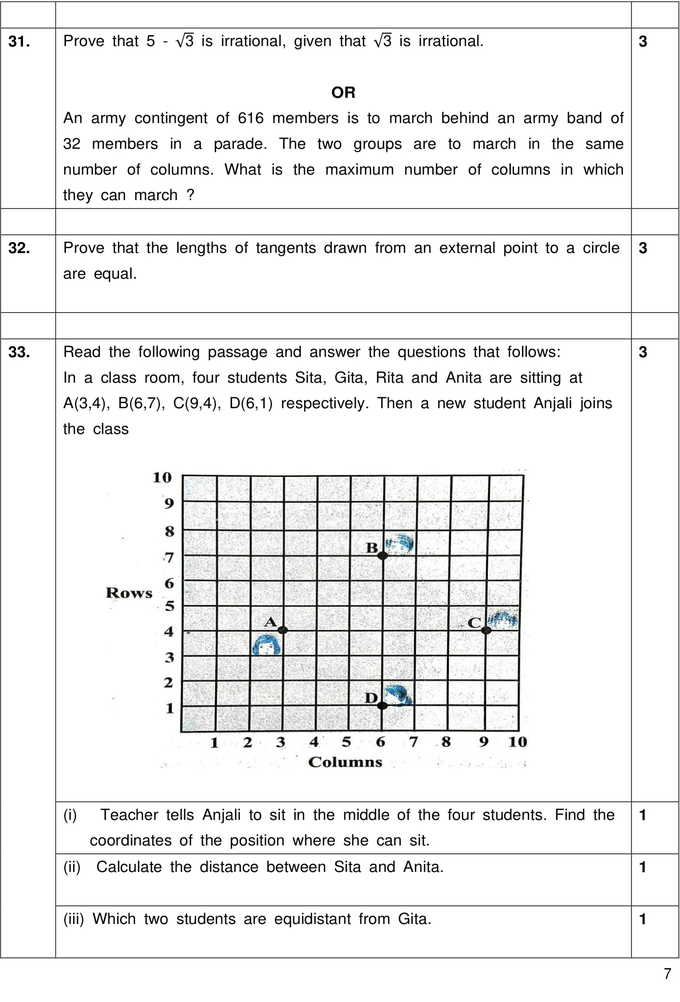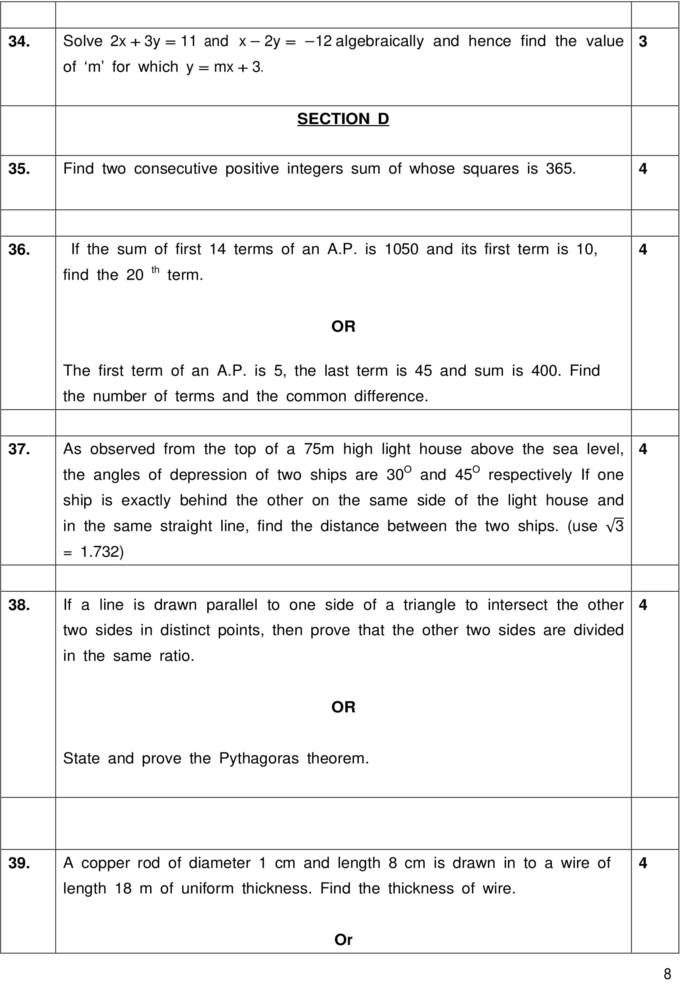## CBSE Math (Basic) Sample Paper 2020| Class 10th

Time Allowed: 3 Hrs
Maximum Marks: 80 Marks

General Instructions:
a) All questions are compulsory
b) The question paper consists of 40 questions divided into four sections A, B, C & D.
c) Section A comprises of 20 questions of 1 mark each. Section B comprises of 6 questions of 2 marks each. Section C comprises of 8 questions of 3 marks each.Section D comprises 6 questions of 4 marks each.
d) There is no overall choice. However internal choices have been provided in two questions of 1 mark each, two questions of 2 marks each, three questions of 3 marks each and three questions of 4 marks each. You have to attempt only one of the alternatives in all such questions.
e) Use of calculators is not permitted.Solutions to CBSE Math Basic Sample Paper 2020 Class 10th